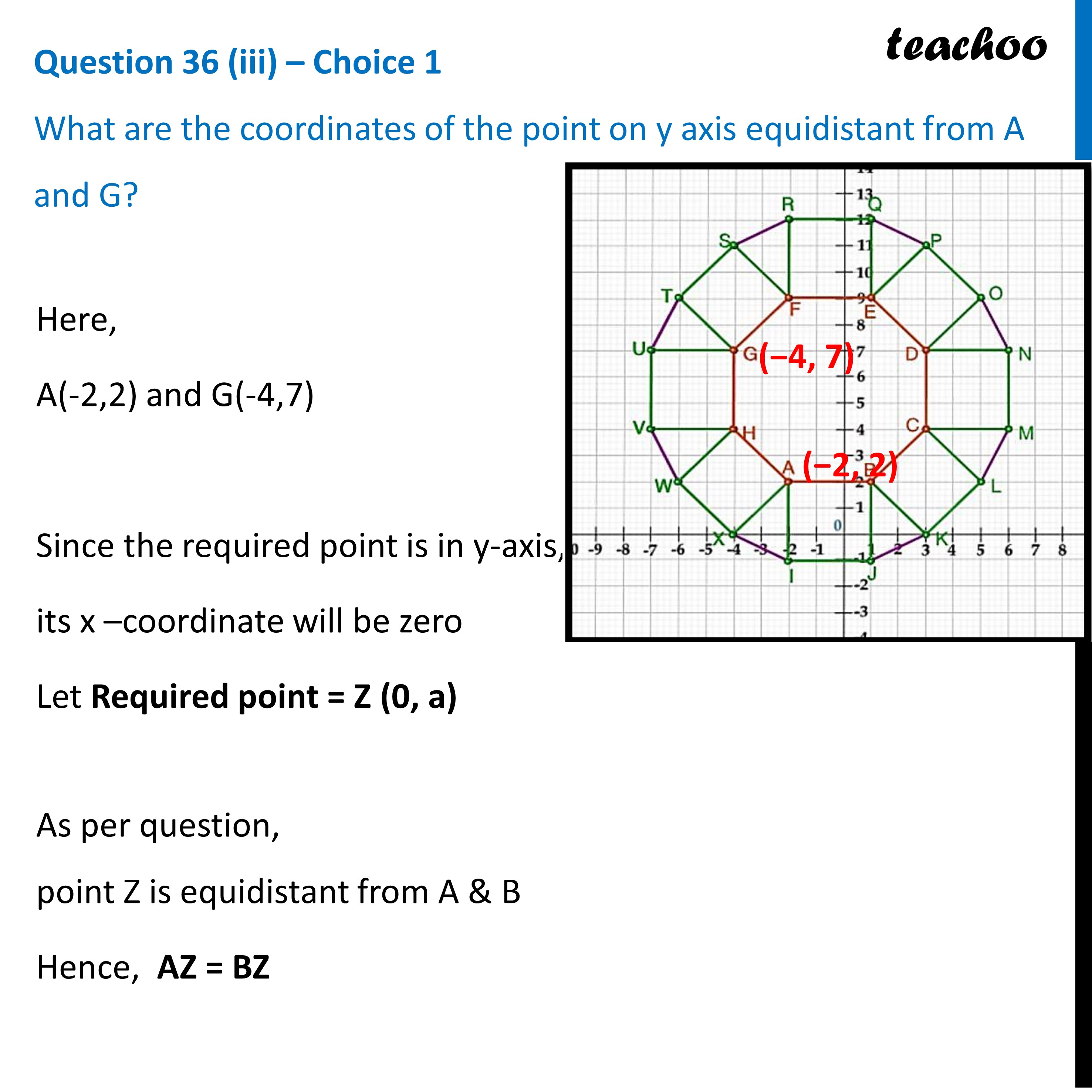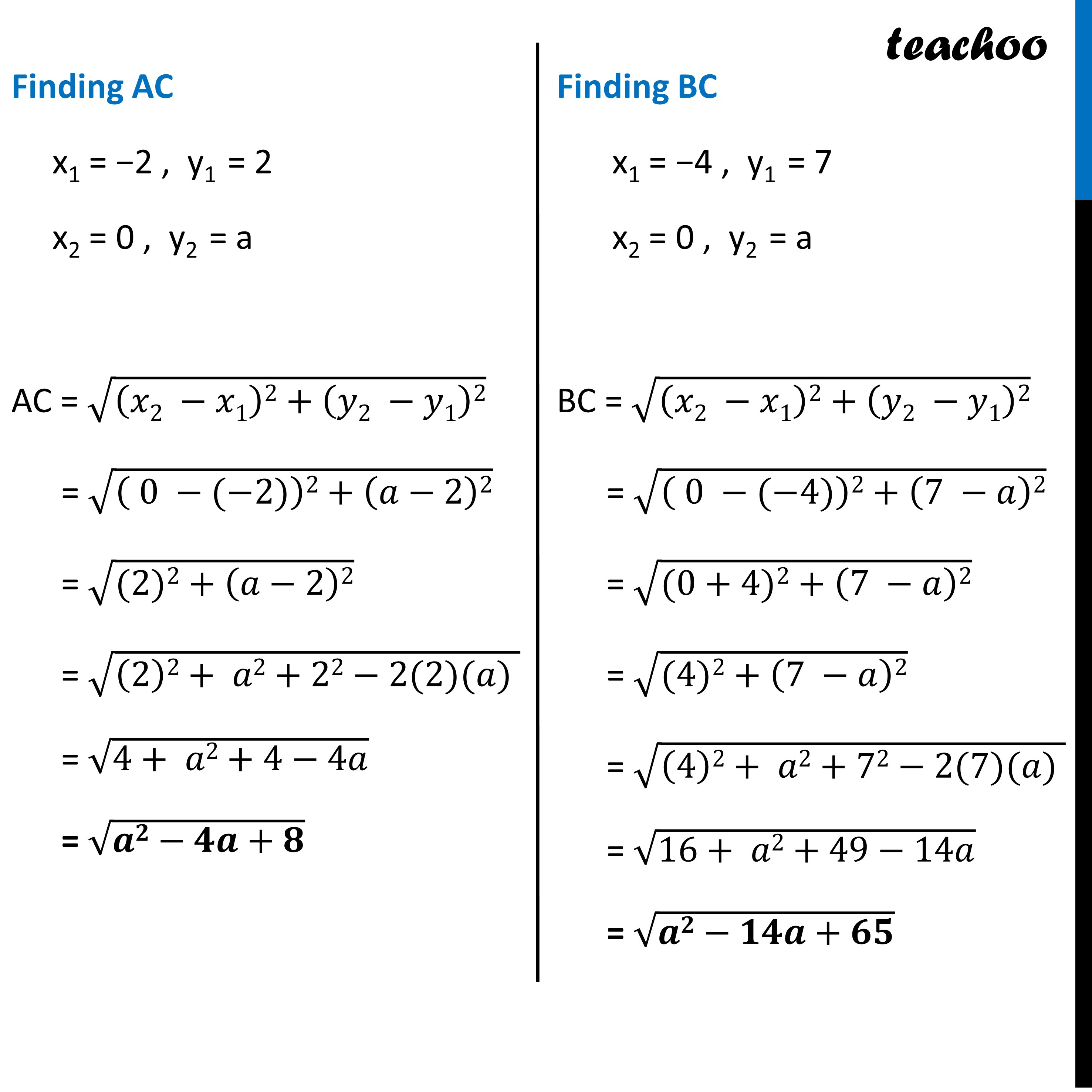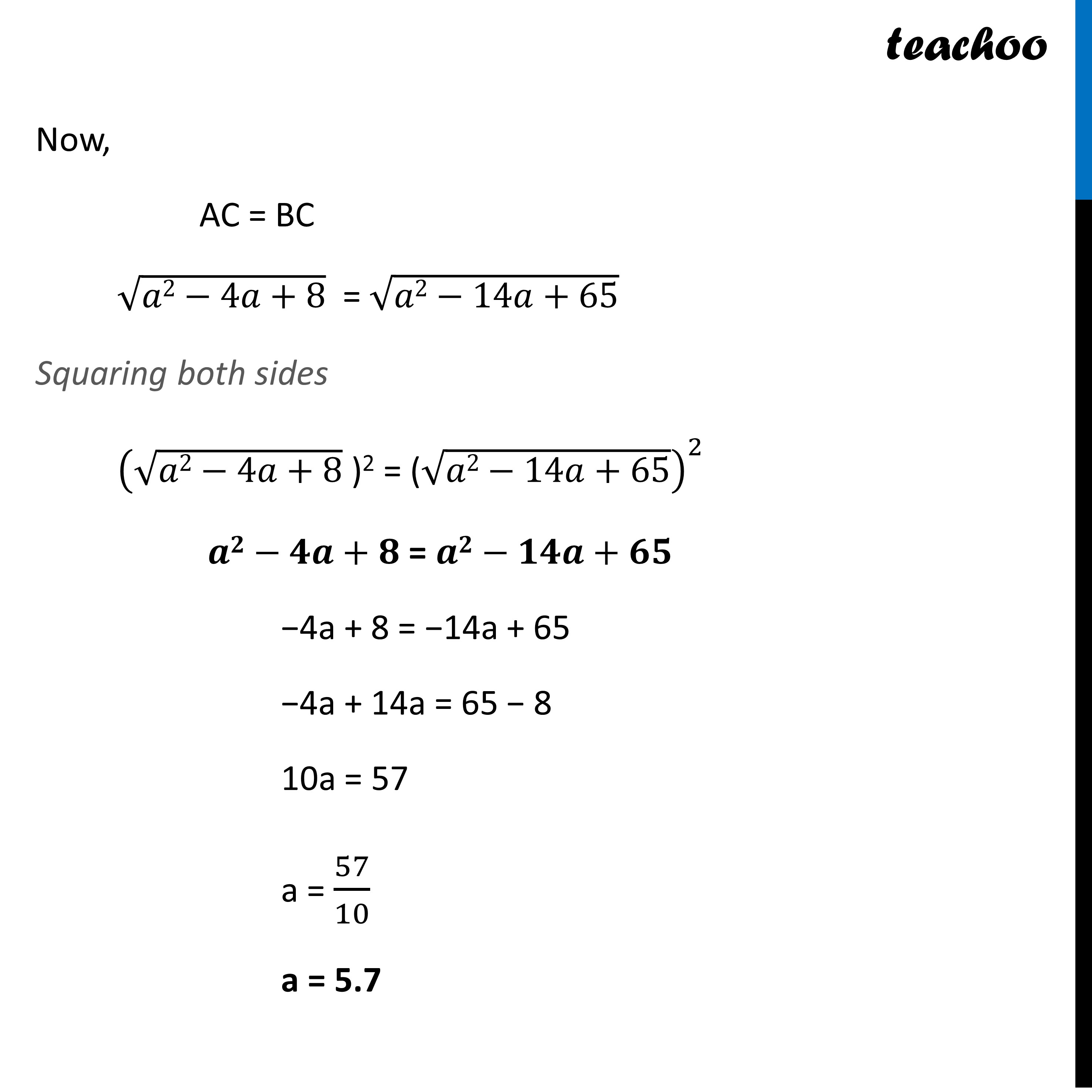CBSE Class 10 Sample Paper for 2023 Boards - Maths Standard

Class 10
Solutions of Sample Papers for Class 10 Boards

## (iii)What are the coordinates of the point on y axis equidistant from A and G?'

Learn in your speed, with individual attention - Teachoo Maths 1-on-1 Class

### Transcript

Question 36 (iii) – Choice 1 What are the coordinates of the point on y axis equidistant from A and G? Here, A(-2,2) and G(-4,7) Since the required point is in y-axis, its x –coordinate will be zero Let Required point = Z (0, a) As per question, point Z is equidistant from A & B Hence, AZ = BZ Finding AC x1 = −2 , y1 = 2 x2 = 0 , y2 = a AC = √((𝑥2 −𝑥1)2+(𝑦2 −𝑦1)2) = √(( 0 −(−2))2+(𝑎−2)2) = √((2)2+(𝑎−2)2) = √((2)2+ 𝑎2+22−2(2)(𝑎) ) = √(4+ 𝑎2+4−4𝑎) = √(𝒂𝟐−𝟒𝒂+𝟖) Finding BC x1 = −4 , y1 = 7 x2 = 0 , y2 = a BC = √((𝑥2 −𝑥1)2+(𝑦2 −𝑦1)2) = √(( 0 −(−4))2+(7 −𝑎)2) = √((0+4)2+(7 −𝑎)2) = √((4)2+(7 −𝑎)2) = √((4)2+ 𝑎2+72−2(7)(𝑎) ) = √(16+ 𝑎2+49−14𝑎) = √(𝒂𝟐−𝟏𝟒𝒂+𝟔𝟓) Now, AC = BC √(𝑎2−4𝑎+8) = √(𝑎2−14𝑎+65) Squaring both sides (√(𝑎2−4𝑎+8) " )2 = (" √(𝑎2−14𝑎+65))^2 𝒂𝟐−𝟒𝒂+𝟖 = 𝒂𝟐−𝟏𝟒𝒂+𝟔𝟓 −4a + 8 = −14a + 65 −4a + 14a = 65 − 8 10a = 57 a = 57/10 a = 5.7 Hence the required point is C(0, a) = (0, 9)